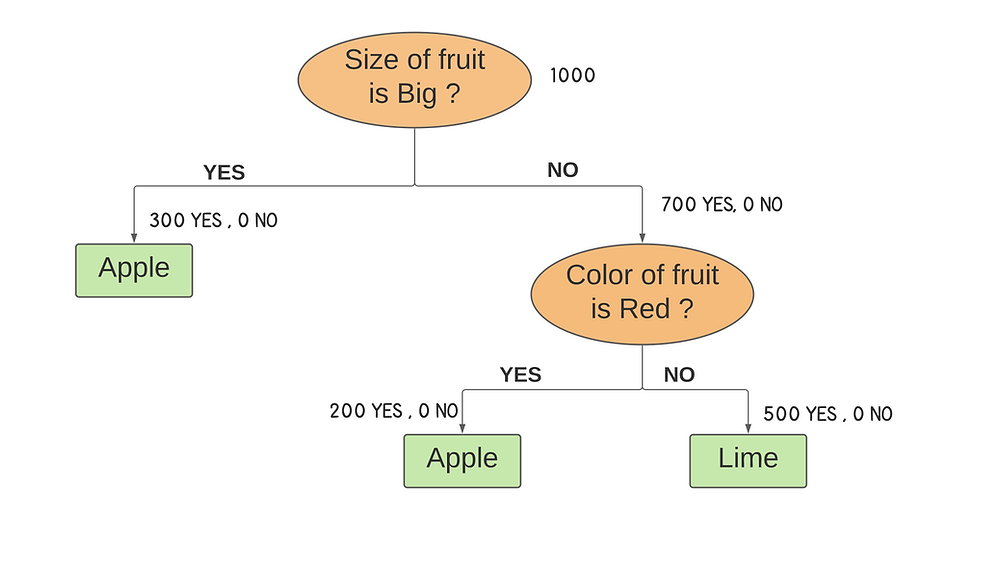Search

# Decision Trees - What , How and Why?### What is a Decision Tree?

Decision Tree is the most powerful and popular tool for Classification and Prediction.

Decision Tree is a tree shaped diagram used to determine a course of action. Each branch of the tree represents a possible decision, occurrence or reaction.

In a Decision Tree, the data is continuously split according to a certain parameter or feature. Splitting is a process of dividing the source set into subsets based on an attribute value test. This process is repeated on each derived subset in a recursive manner. The recursion is performed during the training process until only homogenous nodes are left.

And it is the only reason why a decision tree can perform so well. Therefore, Node splitting, is the process of dividing a node into multiple sub-nodes to create relatively pure nodes.

The figure below demonstrates a simple decision tree that can be used to classify a fruit as an apple or a lime based upon "features" of the fruit like color and size.The oval shapes in the tree, where the questions about features are asked, are called Nodes. The first node in a decision tree is called the Root Node (Color of the fruit) .The lines carrying the information about the features between the nodes are called Branches (Red/Green/Big/Small). At the end of a branch, comes a node (which might split into more branches ) or a Leaf Node (which doesn't split further into branches). The Leaf Nodes in the above example are Apple and Lime.

Suppose there's a fruit orchard that grows red apples, green apples and green limes and needs to be packed into cartons before leaving the orchard. But the problem is the fruits are all mixed up . We can build a machine that can segregate the fruits according to the features into the respective cartons.

So, we have to start training the machine. So, how can we start ?

We can ask for 1000 fruits to train the machine which contains 500 apples and 500 limes.So, what can we infer from the data?

Out of the 500 apples,

1. 200 apples are big and red.

2. 200 apples are small and red.

3. 100 apples are big and green.

and all 500 limes are small and green.

So, the machine tries to build a decision tree classifier.

### How to build a Decision Tree?

So, how can we split the data into subsets?

We can actually build 2 Decision Trees - a) with Color as the Root node and

b) with Size as the Root node.

How can we decide which DT will yield the best result?

For that , we have to actually learn about 2 terms called Entropy and Information Gain.

Entropy is a measure of disorder or uncertainty and the goal of machine learning models and Data Scientists in general is to reduce uncertainty. Entropy is measured between 0 and 1.How can we quantify the quality of a split? - by calculating Information Gain.

Information Gain is calculated for a split by subtracting the weighted entropies of each branch from the original entropy.When training a Decision Tree using these metrics, the best split is chosen by maximising Information Gain.

Decision Tree 1 - Splitting according to ColorBefore the Split , we have 400 Red fruits and 600 Green fruits . So,Decision Tree 2 - Splitting according to Size:Before the Split , we have 300 Big fruits and 700 Small fruits . So,So to conclude,

Information Gain of Tree (Split by Color ) - 0.5807

Information Gain of Tree (Split by Size ) - 0.277

So, the split by the feature Color has more Information Gain, meaning the quality of the split is higher( more Entropy is removed ), if we select Color as the Root Node. So, splitting by Color first is recommended.

Again, the Steps to split a decision tree using Information Gain:

1. For each split, individually calculate the entropy of each child node

2. Calculate the entropy of each split as the weighted average entropy of child nodes

3. Select the split with the lowest entropy or highest information gain

4. Until you achieve homogeneous nodes, repeat steps 1-3.

### Why use a Decision Tree?

• The main advantage of decision trees is how easy they are to interpret. While other machine Learning models are close to black boxes, decision trees provide a graphical and intuitive way to understand what our algorithm does.

• Decision trees require relatively little effort from users for data preparation.

• Nonlinear relationships between parameters do not affect tree performance.

• Can handle both numerical and categorical data. Can also handle multi-output problems.

• They can be used for predicting missing values, suitable for feature engineering techniques.